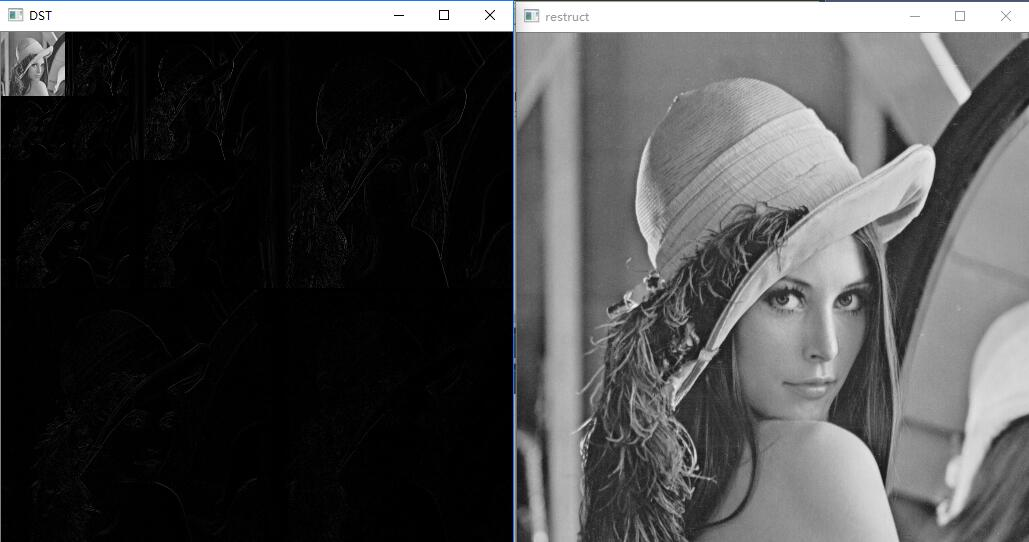2015-10-27 18:13:44 robin1987z 阅读数 11507
• ###### MATLAB图像处理

全面系统的学习MATLAB在图像处理中的应用

19908 人正在学习 去看看 魏伟
##### 小波变换原理

所谓的小波的小是针对傅里叶波而言，傅里叶波指的是在时域空间无穷震荡的正弦（或余弦波）。

相对而言，小波指的是一种能量在时域非常集中的波，它的能量有限，都集中在某一点附近，而且积分的值为零，这说明它与傅里叶波一样是正交波。

举一些小波的例子：众所周知，图像的傅里叶变换是将图像信号分解为各种不同频率的正弦波。同样，小波变换是将图像信号分解为由原始小波位移和缩放之后的一组小波。

小波在图像处理里被称为图像显微镜，原因在于它的多分辨率分解能力可以将图片信息一层一层分解剥离开来。剥离的手段就是通过低通和高通滤波器，

1.用低通滤波器与原始像素矩阵做卷积得：[8 10 12 15 12 14 12 5]/sqrt(2)

2.用高通滤波器与原始像素矩阵做卷积得：[-4 2 -4 1 2 -4 6 1]/sqrt(2)

1.对L数组插值得：[0 10 0 15 0 14 0 5]/sqrt(2)

2.对H数组插值得：[0 2 0 1 0 -4 0 1]/sqrt(2)

##### 基于小波变换的图像压缩2005-09-01 15:47:00 byxdaz 阅读数 4885
• ###### MATLAB图像处理

全面系统的学习MATLAB在图像处理中的应用

19908 人正在学习 去看看 魏伟

［ 作者：佚名    转贴自：南京大学      更新时间：2004-5-27    文章录入：johnchen］

一：引言 本文从二维小波理论出发，对其在图像处理的应用上进行了一些分析和处理，力图反映出小波分析在图像处理方面有着其独特的特点。本文就以下几点进行阐述：

①小波基本概念

②图像压缩

③图像消噪

④图象增强

⑤图象平滑处理

二：小波基本概念

图像数据往往存在各种信息的冗余、如空间冗余、信息熵冗余 、视觉冗余 和结构冗余等等。所谓压缩就是去掉各种冗余，保留对我们有用的信息。图像压缩的过程常称为编码。相对的，图像的恢复当然就是解码了。

图像压缩的方法通常可分为有失真编码和无失真编码两大类：

无失真编码方法如改进的霍夫曼编码。

有失真编码方法的还原图像较之原始图像存在着一些误差，但视觉效果是可以接受的。常见的方法有预测编码、变换编码、量化编码、信息熵编码、分频带编码和结构编码等等。

而将小波分析引入图像压缩的范畴也是一个重要的手段，并且有着它自己的特点。它的特点在于压缩比高、压缩速度快，压缩后能保持信号与图像的特征基本不变，且在传递过程中可以抗干扰等等。

下面我们就举一个粒子来说明怎样用小波分析进行图像压缩。

例如现在有一个二维图像（文件名为），我们利用二维小波分析来进行图像压缩。

clear

%装入图像

%显示图像

syms X;

subplot(221);

image(coast);

colormap(map)

title('原始图像');

axis square

disp('压缩前图像X的大小');

whos('coast')

%对图像用小波进行层小波分解

[c,s]=wavedec2(X,2,'bior3.7');

%提取小波分解结构中的一层的低频系数和高频系数

cal=appcoef2(c,s,'bior3.7',1);

%水平方向

ch1=detcoef2('h',c,s,1);

%垂直方向

cv1=detcoef2('v',c,s,1);

%斜线方向

cd1=detcoef2('d',c,s,1);

%各频率成份重构

a1=wrcoef2('a',c,s,'bior3.7',1);

h1=wrcoef2('h',c,s,'bior3.7',1);

v1=wrcoef2('v',c,s,'bior3.7',1);

d1=wrcoef2('d',c,s,'bior3.7',1);

c1=[a1,h1;v1,d1];

%显示分频信息

subplot(222);

image(c1);

axis square;

title ('分解后低频和高频信息');

%进行图像压缩

%保留小波分解第一层低频信息

%首先对第一层信息进行量化编码

ca1=appcoef(c,s,'bior3.7',1);

ca1=wcodemat(ca1,440,'mat',0);

%改变图像高度并显示

ca1=0.5*ca1;

subplot(223);

image(ca1);

colormap(map);

axis square;

title('第一次压缩图像');

disp('第一次压缩图像的大小为：');

whos('ca1')

%保留小波分解第二层低频信息进行压缩

ca2=appcoef2(c,s,'bior3.7',2);

%首先对第二层信息进行量化编码

ca2=wcodemat(ca2,440,'mat',0);

%改变图像高度并显示

ca2=0.25*ca2;

subplot(224);

image(ca2);

colormap(map);

axis square;

title('第二次压缩图像');

disp('第二次压缩图像的大小为：');

whos('ca2')

Name
Size
Bytes
class

X
256×256
524288
Double array

Ca1
135×135
145800
Double array

Ca2
75×75
45000
Double array

上面的保留原始图像中低频信息的压缩办法只是一种最简单的压缩办法。它不需经过其他处理即可获得较好的压缩效果。当然，对于上面的例子我们还可以只提取小波分解的第三、第四层的低频信息。从理论上说，我们可以获得任意压缩比的压缩图像。只不过在对压缩比和图像质量都有较高要求时，它就不如其他编码方法了。

Clear;

%装入图形信号

%显示图像

subplot(221);

image(X);

colormap(map);

title('原始图像');

disp('压缩前图像的大小');

whos('X');

axis square;

%对图像进行压缩

%对图像用db3小波进行二层小波分解

[c,s]=wavedec2(X,5,'db3');

[thr,sorh,keepapp]=ddencmp('cmp','wv',X);

[Xcomp,cxc,lxc,perf0,perfl2]=wdencmp('gbl',c,s,'db3',5,thr,sorh,keepapp);

%将压缩后的图像于原始图像相比较

subplot(222);

image(Xcomp);

colormap(map);

title(' 压缩后的图像');

disp('压缩后图像的大小');

whos('Xcomp')

%显示有关参数

disp('小波分解系数中值为0的系数个数百分比');

disp(perf0);

disp('压缩后剩余能量百分比');

disp(perfl2);

总之，是事无绝对。一种压缩图像的方法不可能尽善尽美。要想很好的进行图像的压缩，就需要综合的利用多种其他技术，特别是数据编码和解码算法。

2016-02-22 16:01:31 zizi7 阅读数 9768
• ###### MATLAB图像处理

全面系统的学习MATLAB在图像处理中的应用

19908 人正在学习 去看看 魏伟

## 2. 小波变换基本原理## 4. 代码

Matlab的可以参考晨宇思远博客的小波系列博文

C的可以参考http://eeweb.poly.edu/~onur/source.html，里面包含了小波及小波包分解重构的实现## 5. 参考

 晨宇思远博客小波变换系列

 清华小波变换课件

2018-05-05 17:22:19 Qwilfish 阅读数 959
• ###### MATLAB图像处理

全面系统的学习MATLAB在图像处理中的应用

19908 人正在学习 去看看 魏伟

https://blog.csdn.net/qq_20823641/article/details/51829981

```#include"stdafx.h"
#include<opencv2\opencv.hpp>
#include<iostream>
using namespace cv;
int thundershock() {
Mat dst;
int Width = src.cols;
int Height= src.rows;
//小波分解次数
int depth = 1;//
int depthcount = 1;
//改变数据格式防止溢出
Mat tmp = Mat::zeros(src.size(), CV_32FC1);
Mat  wavelet = Mat::zeros(src.size(), CV_32FC1);
Mat  imgtmp = src.clone();
imgtmp.convertTo(imgtmp, CV_32FC1);
//执行小波变换
while (depthcount <= depth) {
Width = src.cols / pow(2,depthcount-1);
Height = src.rows/pow(2,depthcount-1);
for (int i = 0; i < Height; i++) {
for (int j = 0; j < Width / 2; j++) {
tmp.at<float>(i, j) = (imgtmp.at<float>(i, 2 * j) + imgtmp.at<float>(i, 2 * j + 1)) / 2;
tmp.at<float>(i, j + Width / 2) = (imgtmp.at<float>(i, 2 * j) - imgtmp.at<float>(i, 2 * j + 1)) / 2;
}

}
for (int i = 0; i < Height / 2; i++) {
for (int j = 0; j < Width; j++) {
wavelet.at<float>(i, j) = (tmp.at<float>(2 * i, j) + tmp.at<float>(2 * i + 1, j)) / 2;
wavelet.at<float>(i + Height / 2, j) = (tmp.at<float>(2 * i, j) - tmp.at<float>(2 * i + 1, j)) / 2;
}
}
imgtmp = wavelet;
depthcount++;
}
namedWindow("DST", WINDOW_AUTOSIZE);
convertScaleAbs(wavelet, dst);

imshow("DST", dst);
//反变换
while (depthcount > 1) {

for (int i = 0; i < Height / 2; i++) {
for (int j = 0; j < Width; j++) {
tmp.at<float>(2*i, j) = wavelet.at<float>( i, j) + wavelet.at<float>(i+Height/2,j);
tmp.at<float>(2*i+1, j) = wavelet.at<float>( i, j) - wavelet.at<float>(i + Height/2, j);
}
}
for (int i = 0; i < Height; i++) {
for (int j = 0; j < Width / 2; j++) {
imgtmp.at<float>(i, 2*j) = tmp.at<float>(i,  j) + tmp.at<float>(i,  j + Width/2);
imgtmp.at<float>(i, 2*j+1) = tmp.at<float>(i,  j) - tmp.at<float>(i,  j + Width/2);
}
}
depthcount--;
wavelet = imgtmp;
Height *= 2;
Width *= 2;

}
namedWindow("restruct", WINDOW_AUTOSIZE);
convertScaleAbs(imgtmp, imgtmp);

imshow("restruct", imgtmp);
waitKey();
imwrite("haar.jpg", dst);
return 0;
}

```
`附执行结果：`2005-09-01 15:49:00 byxdaz 阅读数 5318
• ###### MATLAB图像处理

全面系统的学习MATLAB在图像处理中的应用

19908 人正在学习 去看看 魏伟

［ 作者：佚名    转贴自：南京大学       更新时间：2004-5-27    文章录入：johnchen ］

四：图像消噪

图像消噪方法的一般说明

（1）       二维信号的小波分解 。选择一个小波和小波分解的层次N, 然后计算信号s到第N层的分解。

（2）       对高频系数进行阀值量化。对于从一到N的每一层，选择一个阀值，斌对着一层的高频系数进行软阀值化处理。

（3）       二维小波的重构。根据小波分解的第N层的低频系数和经过修改的从第1层到第N层的各层高频系数，来计算二维信号的小波重构。

编程

subplot(221);

image(X);

colormap(map);

title('原图 ');

axis square;                      ％画出原图象

init=2055615866;

randn('seed',init)

x=X+38*randn(size(X));

subplot(222);

image(x);

colormap(map);

title('含噪声图象 ');

axis square;　　　　　　　　　　％画出含噪声图象

[c,s]=wavedec2(x,2,'sym4');

a1=wrcoef2('a',c,s,'sym4',1); ％第一次低通滤波消噪

subplot(223);

image(a1);

title('第一次消噪后图象 ');

axis square;                        ％画出第一次低通滤波消噪后图象

a2=wrcoef2('a',c,s,'sym4',2); ％第二次低通滤波消噪

subplot(224);

image(a2);

title('第二次消噪后图象 ');

axis square;　　　　　　　　　　　％画出第二次低通滤波消噪后图象

五：图象增强

说明

[分析]由于图象经二维小波分解后，图象的轮廓主要体现在低频部分，而细节部分则体现子高频部分，因此，可以通过对低频分解系数进行增强处理，对高频分解系数进行衰减处理，即可以达到图象增强的作用。

具体处理过程如下程序：

subplot(121);

image(X);

colormap(map);

title(‘原始图象‘)；

axis square;　　　　　　　　　　％画出原图象

[c,s]=wavedec2(X,2,’sym4’);    %进行二层小波分解

sizec=size(c);　　　　　　　　　％处理分解系数，突出轮廓，弱化细节

for I =1:sizec(2)

if(c( I )>350)

c( I )=2*c( I );

else

c( I )=0.5*c( I );

end

end

xx=waverec2(c,s,’sym4’);　　　　％分解系数重构

subplot(122);

image(xx);

title(‘增强图象‘)

axis square;　　　　　　　　　　％画出增强图像

说明

X1=X;map1=map;

subplot(221);

image(X1);

colormap(map1);

title(‘woman’);

axis square                                 %画出woman图像

X2=X;map2=map;

for I =1:256

for j=1:256

if(X2(I, j)>100)

X2(I, j)=1.2*X2(I, j);

else

X2(I, j)=0.5*X2(I, j);

end

end

end

subplot(222);

image(X2);

colormap(map2);

title(‘wbarb’);

axis square　　　　　　　　　　　　　　　　　%画出wbarb图像

[cl,sl]=wavedec2(X1,2,’sym4’);

sizec1=size(c1);

for I=1:sizec1(2)

c1( I )=1.2*c1( I );

end

[c2,s2]=wavedec2(X2,2,’sym4’);

c=c1+c2;

c=0.5*c;

xx=waverec2(c,s,’sym4’);

subplot(223);image(xx);

title(‘融合图象‘)；

axis square                                   %画出融合后的图像

说明

编程

[分析]这是一个图象平滑处理问题。首先，对图象在频域内进行增强，然后在空域内加入较大的白噪声。通过对含噪图象进行平滑处理，即可以使含噪图象具有较好的平滑效果。具体处理过程如下：

X1=X;

map1=map;

subplot(221);

image(X1);

colormap(map1);

title('woman');

axis square                               ％画出原图

[c,s]=wavedec2(X,2,’sym4’);                               ％二层分解小波信号

sizec=size( c );

for I= 1:sizec(2)　　　　　　　　　　　　％频域里增强图像

if(c( I )>350)

c( I )=1.3*c( I );

else

d( I )=0.5*c( I );

end

end

xx=waverec(c,s,’sym4’);　　　　　　　　％系数重构

init=2788605826;　　　　　　　　　　　％加入噪声

rand(‘seed’,init);

xx=xx+68*(rand(size(xx)));

subplot(221);image(xx);

title(‘增强的含噪图象‘)；

axis square;

for I=2:1:255　　　　　　　　　　　　　　％中值滤波

for j=2:1:255

temp=0;

for m=1:3

for n=1:3

temp=temp+xx(I+m-2,j+n-2);

end

end

temp=temp/9;

xx(I, j)=temp;

end

end

colormap(map);

subplot(222);

image(xx);

axis square;

title(‘平滑后的图象‘)；

axis square                               %画出平滑后图像

功能

dwt2
单层二维小波分解

dwtper2
单层二为离散小波变换

wavedec2
多层二维小波分解

idwt2
单层二微小波重构

idwper2
单层二维小波分解

waverec2
多层二维小波重构

upwiev2
二维小波分解的单层重构

wrcoef2
二维小波分解系数单支重构

upcoef2
二维小波分解的直接重构

detcoef2
提取二微小波分解高频系数

appcoef2
提取二维小波分解低频系数

wthresh
进行软阈值或硬阈值处理

wthcoef2
二维信号的小波系数阈值处理

ddencmp
获取在消噪或压缩过程中的默认值阈值

wdencmp
用小波进行信号的消噪和压缩